nextnano.com  GUI: nextnanomat  Tool: nextnano++  Tool: nextnano³  Tool: nextnano.QCL  Download | Search | Copyright | News | Publications  * password protected nextnano³ software1D Mobility in 2DEGs

# nextnano3 - Tutorial

## Mobility in two-dimensional electron gases (2DEGs)

Authors: Stefan Birner

If you want to obtain the input files that are used within this tutorial, please contact stefan.birner@nextnano.de.
``` -> 1DInSb_mobility_ShaoFig3.in -> 1DGaAs_mobility_WalukiewiczFig2.in -> 1DGaAs_mobility_WalukiewiczFig3.in -> 1DInGaAs_mobility_WalukiewiczFig8.in -> 1DGaN_mobility_WalukiewiczFig4.in -> 1DGaN_mobility_WalukiewiczFig5.in  ```

## Mobility in two-dimensional electron gases (2DEGs)

### a) Mobility in delta-doped InSb quantum wells

`-> 1DInSb_mobility_ShaoFig3.in`

This tutorial is based on the following paper:

[Shao]
Carrier Mobilities in delta-doped Heterostructures
Y. Shao, S.A. Solin, L.R. Ram-Mohan
(2006)
arXiv:cond-mat/0602140

Our implementation is based on the equations that are given in this paper (with the exception of Eq. (3.5) where we added a factor of 1 / (4 pi) because of SI units).

We calculate the mobility in a 40 nm InSb quantum well that is surrounded by and strained with respect to Al0.15In0.85Sb barriers.
At z =` -`20 nm, there is a delta-doping layer with a sheet doping density of 1 * 1012 cm-2.
The delta-doping layer is separated from the InSb QW by a 40 nm Al0.15In0.85Sb spacer layer.
```  quantum-well-width          = 40d0 ! [nm]    [Shao] Fig. 3  spacer-width                = 40d0 ! [nm]    [Shao] Fig. 3  remote-doping-sheet-density = 1d12 ! [cm^-2] [Shao] Fig. 3  ```

We calculate all properties for different temperatures. This can be done as follows:
```  \$global-parameters   lattice-temperature               = 1d0   ! ```start value``` T = 1 [K]   temperature-sweep-active          = yes   ! 'yes' / 'no'   temperature-sweep-step-size       = 10d0  ! ```increase temperature each time by``` T = 10 [K]   temperature-sweep-number-of-steps = 31    ! ```increase temperature` 31 `times```   data-out-every-nth-step           = 1     ! ```output all data for every temperature sweep```  \$end_global-parameters ```All output files are labelled with an index, starting from zero, that refers to each individual temperature sweep:` .../..._ind000....dat`
Here, the index runs from 0 (`000`) to 30 (`030`), i.e.``` 31 ```output files for each property in total.

We note that band gaps and lattice constants depend on temperature. This is taken into account automatically for each temperature sweep.

The following figure shows the conduction band edge, the Fermi level and the square of the lowest two electron wave functions at T = 1 K.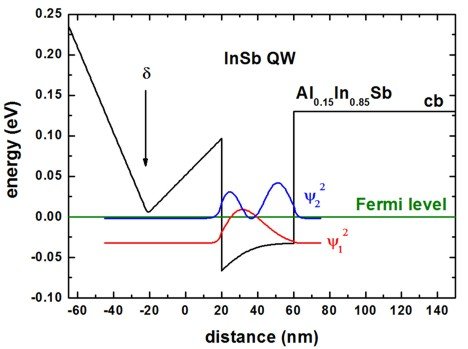To plot such a figure, the following output files are needed:
```- band_structure/cb1D_001_ind000.dat:   ```1st  column: distance` [nm]`
`  `2nd column: conduction band edge at the Gamma point``` [eV]   ```Note: The index '`ind000`' refers to the temperature sweep. Here, index 0 means T = 1 K.``` - current/fermi1Del_ind000.dat:   ```1st  column: distance``` [nm]   ```2nd column: Fermi level of the electrons``` [eV]   ```In this tutorial, the Fermi level is always equal to 0 eV.``` - Schroedinger_1band/cb001_ind000_qc001_sg001_deg001_dir_Kz001.dat:   ```1st  column: distance` [nm]`
`  `n  columns: n energies of the eigenstates``` [eV]   ```n  columns: n squares of the wave functions (psi2)``` [eV]   ```In the figure, we plotted the columns for psi2 of the two lowest states: psi12, psi22

The following figure shows the sheet electron density as a function of temperature.
We considered the two lowest subbands for calculating the 2DEG density (the spin degeneracy of the subbands is included):
``` 2DEG-sheet-density-number-of-subbands = 2 ```
Our results differ from the results of Fig. 3(b) of the [Shao] paper.
(Some obvious discrepancies are the conduction band offset (We used ~0.15 eV whereas [Shao] used ~0.25 eV.) and the Schottky barrier height.)To plot such a figure, the following output file was used:
```- Monte_Carlo/mobility_TemperatureSweep.dat:   ```1st   column:  temperature` [K]`
`  `last column:  electron sheet density of the lowest subband(s)` [m-2]`

The following figure shows the calculated 2DEG mobility as a function of temperature.
The relevant data can be found in this file:
```- Monte_Carlo/mobility_TemperatureSweep.dat:   ```1st  column:  temperature` [K]`
`  `2nd column:  total mobility``` [m2/Vs]   ```3rd  column:  mobility due to ionized        impurity scattering``` [m2/Vs]   ```4th  column:  mobility due to background impurity scattering``` [m2/Vs]   ```5th  column:  mobility due to deformation potential acoustic phonon scattering``` [m2/Vs]   ```6th  column:  mobility due to polar optic (LO)                      phonon scattering` [m2/Vs]`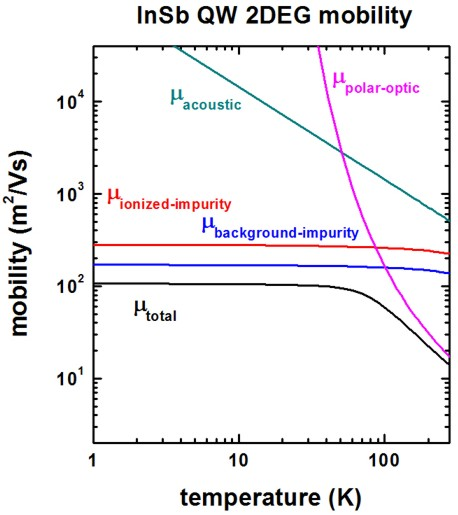We included the following scattering mechanisms:
``` ionized-impurity-scattering     = yes ! [Shao] ```(including remote and background ionized impurity scattering)```  acoustic-phonon-scattering      = yes ! [Shao]  polar-optical-phonon-scattering = yes ! [Shao]```
``` alloy-scattering                = no  ! [Shao]```

We now discuss the agreement/disagreement compared to Fig. 3(a) of the [Shao] paper.
`- `The mobility due to acoustic phonon scattering is in excellent agreement.
`- `The mobility due to polar optic LO phonon scattering is in excellent agreement if one takes into
account that [Shao] forgot to include the factor of 1/(4 pi) due to SI units.
`- `The mobility due to ionized and background impurity scattering differs significantly.
It seems that the disagreement is not only due to the different sheet density that has been used.
We used the following value:
```  impurity-background-doping-concentration = 5d15 ! [cm^-3] [Shao] Fig. 3```

The following InSb material parameters have been used:

``` conduction-band-masses         = 0.0135d0 0.0135d0 0.0135d0 ! [m0] [Shao]                                   ...                        !```
``` static-dielectric-constants    = 16.82d0  16.82d0  16.82d0  ! [Shao] epsilon(0)  optical-dielectric-constants   = 15.7d0                     ! [Shao] epsilon(infinity)  LO-phonon-energy               = 0.025d0                    ! [eV] [Shao] (optical phonon energy)  mass-density                   = 5.79d3                     ! [kg/m^3]  [Shao]  sound-velocity                 = 3.7d3                      ! [m/s]     [Shao]  acoustic-deformation-potential = 7.2d0                      ! [eV]      [Shao]```

### b) Mobility in doped GaAs quantum wells

`-> 1DGaAs_mobility_WalukiewiczFig2.in - `(Experiment of Hiyamizu et al.)``` -> 1DGaAs_mobility_WalukiewiczFig3.in - ```(Experiment of DiLorenzo et al.)

Here, we test our algorithm to results on GaAs 2DEGs of another publication.
We note that our algorithm is suitable for delta-doped 2DEGs but the GaAs examples are not delta-doped.

This tutorial is based on the following paper:

[Walukiewicz]
Electron mobility in modulation-doped heterostructures
W. Walukiewicz, H.E. Ruda, J. Lagowski, H.C. Gatos
Physical Review B 30, 4571 (1984)

The experimental data is based on:

[Walukiewicz, Fig. 2]: [Hiyamizu]
Improved Electron Mobility Higher than 10^6 cm^2/Vs in Selectively Doped GaAs/N-AlGaAs Heterostructures grown by MBE
S. Hiyamizu, J. Saito, K. Nanbu
Japan. J. Appl. Phys. 22, L609 (1983)

[Walukiewicz, Fig. 3]: [DiLorenzo]
Material and device considerations for selectively doped heterojunction transistors
J.V. DiLorenzo, R. Dingle, M. Feuer, A.C. Gossard, R. Hendel, J.C.M. Hwang, A. Kastalsky, V.G. Keramidas, R.A. Kiehl, P. O'Connor
IEEE-IEDM (International Electron Devices Meeting) 28, 578 (1982)

 [Walukiewicz, Fig. 2]: [Hiyamizu] [Walukiewicz, Fig. 3]: [DiLorenzo] Conduction band profile and wave functions Conduction band profile and wave functions 20 nm Al0.3Ga0.7As spacer ```spacer-width = 20d0   ! 20 [nm]``` 23 nm Al0.3Ga0.7As spacer ```spacer-width = 23d0   ! 23 [nm]```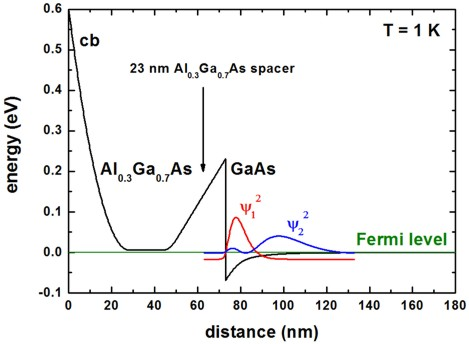2DEG sheet density 2DEG sheet density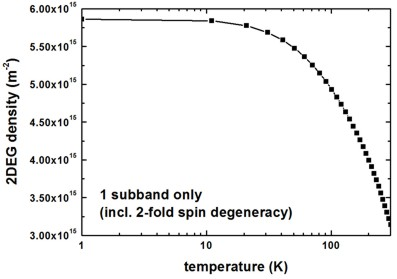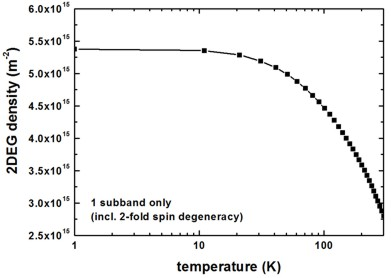Mobility Mobility ```  impurity-background-doping-concentration = 9d13 ! [cm-3]  remote-doping-sheet-density       = 3.5d11      ! [cm-2] ``` (to fit experiment)``` (remote-doping-sheet-density       = 1.948344d11 ! [cm-2])  ```[Walukiewicz] Fig. 2: 8.6 * 1016 [cm-3]``` ==> ```8.6 * 1016 [cm-3]2/3``` = 1.948344d11 (?)``` ``` impurity-background-doping-concentration = 1d15 ! [cm-3] remote-doping-sheet-density              = 1d12 ! [cm-2]  ```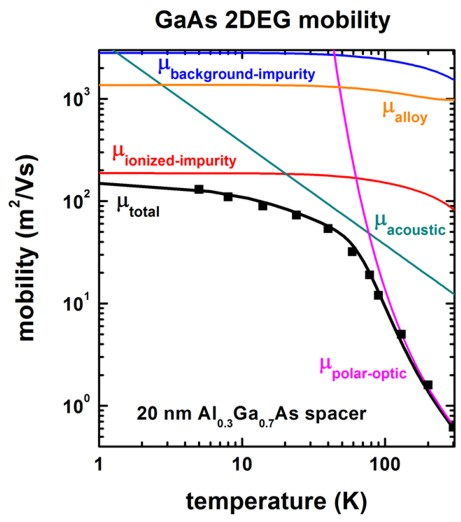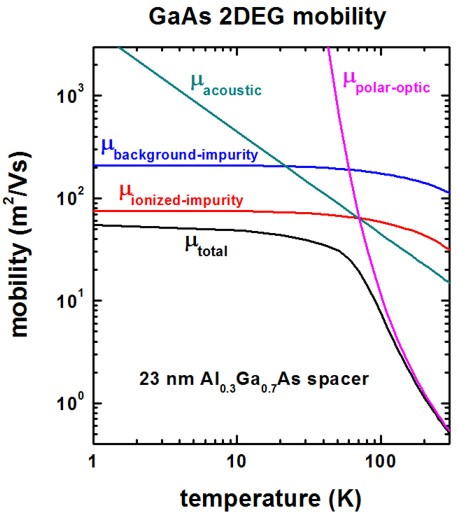Differences with respect to Walukiewicz paper Differences with respect to Walukiewicz paper In Walukiewicz's paper, the background impurity scattering is dominating the ionized impurity scattering. We found the opposite. Walukiewicz used a 2DEG density of 3 * 1011 cm-2. Walukiewicz used a 2DEG density of 2.2 - 3.8 * 1011 cm-2. ```conduction-band-masses = 0.067d0 0.067d0 0.067d0 ! [m0]                          ...                     ! ```a higher value than for bulk because of nonparabolicity Here we used 0.067 as this gives better agreement to the mobility at higher temperatures and this is the usually accepted material parameter for GaAs `quantum-well-width = 13d0 ! [nm] `(13 nm seems to be a better value than 20 nm.) ```alloy-scattering   = yes ``` Alloy scattering is relevant for the part of the wave function that penetrates into the AlGaAs barrier.The squares are experimental values of Fig. 5 in: Improved Electron Mobility Higher than 106 cm2/Vs in Selectively Doped GaAs/N-AlGaAs Heterostructures Grown by MBE S. Hiyamizu et al. Jpn. J. Appl. Phys. 22, L609 (1983) ```conduction-band-masses = 0.076d0 0.076d0 0.076d0 ! [m0] [Walukiewicz]                          ...                     ! ```a higher value than for bulk because of nonparabolicity. This is also the value used by Walukiewicz.   `quantum-well-width = 20d0 ! [nm] `(This value might be too large. See left where 13 nm was used.) ```alloy-scattering   = no ```

The following GaAs material parameters have been used:

``` static-dielectric-constants    = 12.9d0  12.9d0  12.9d0  ! [Walukiewicz] epsilon(0)  optical-dielectric-constants   = 10.9d0                  ! [Walukiewicz] epsilon(infinity)  LO-phonon-energy               = 0.036d0                 ! [eV] [Walukiewicz] (optical phonon energy)  mass-density                   = 5.318d3                 ! [kg/m^3]  [Davies] p. 411  sound-velocity                 = 5.29d3                  ! [m/s]     [X.L. Lei, J. Phys. C 18, L593 (1985)]  acoustic-deformation-potential = 7d0                     ! [eV]      [Walukiewicz]```

Into the equation for the deformation potential acoustic phonon scattering, the quantum well width is an input parameter.
We used a value of 13 nm which corresponds roughly to the extension of the ground state wave function inside the "triangular" QW.

### c) Mobility in doped InGaAs quantum wells

`-> 1DInGaAs_mobility_WalukiewiczFig8.in - `(Experiment of Kastalsky et al.)```  ```

Here, we test our algorithm to results on InGaAs 2DEGs of another publication.
We note that our algorithm is suitable for delta-doped 2DEGs but the InGaAs examples are not delta-doped.

This tutorial is based on the following paper:

[Walukiewicz]
Electron mobility in modulation-doped heterostructures
W. Walukiewicz, H.E. Ruda, J. Lagowski, H.C. Gatos
Physical Review B 30, 4571 (1984)

The experimental data is based on:

[Walukiewicz, Fig. 8]: [Kastalsky]
Two-dimensional electron gas at a molecular beam epitaxial-grown, selectively doped, In0.53Ga0.47As-In0.48Al0.52As interface

A. Kastalsky, R. Dingle, K.Y. Cheng, A.Y. Cho
Applied Physics Letters 41, 274 (1982)

[Kastalsky] and [Walukiewicz] have used In0.48Al0.52As whereas we used In0.52Al0.48As which is lattice matched to InP and In0.53Ga0.47As.
The conduction band profile is shown in the following figure.
Here, two subbands (psi12, psi22) are occupied although our implementation of calculating the mobility is only applicable to one occupied subband.
Note that the 2DEG is located in an alloy, i.e. InGaAs. Thus we expect that alloy scattering has a significant effect on the total mobility.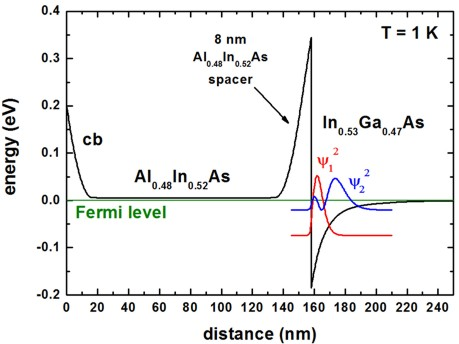The following figure shows the subband density of the first subband and of the first two subbands as a function of temperature.
Inside the mobility algorithm, only the density of the first subband has been considered.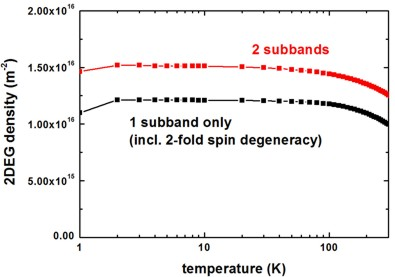The following figure shows the mobility as a function of temperature.
At temperatures below 100 K, the total mobility is dominated by alloy scattering.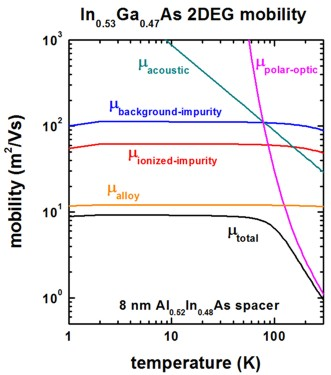Our results for the mobility are in reasonable agreement with Fig. 8 of the paper of [Walukiewicz].

The following parameters have been used:

``` ionized-impurity-scattering              = yes     ! ```(including remote and background ionized impurity scattering)```  acoustic-phonon-scattering               = yes     !  polar-optical-phonon-scattering          = yes     !```
``` alloy-scattering                         = yes     !```
``` quantum-well-width                       = 15d0    ! [nm] ```15 nm seems to be a reasonable approximation for the triangular well
``` spacer-width                             = 8d0     ! [nm]  impurity-background-doping-concentration = 1.0d16  ! [cm-3]  remote-doping-sheet-density              = 1.0d12  ! [cm-2]  2DEG-sheet-density-number-of-subbands    = 1  alloy-disorder-scattering-potential      = 0.60d0  ! [eV] ```InGaAs bulk value [J.R. Hayes et al. (1982)]``` !---------------------------------------------------------------------------- ! In0.53Ga0.47As material parameters !----------------------------------------------------------------------------  mass-density                   = 5.5025d3  ! [kg/m^3] InGaAs            ```[Wen et al., JAP 100, 103516 (2006)]
``` sound-velocity                 = 4.753d3   ! [m/s]    In0.53Ga0.47As ```[Wen et al., JAP 100, 103516 (2006)]
``` acoustic-deformation-potential = 7d0       ! [eV]     InGaAs            ```[Walukiewicz]```  ```

### d) Mobility in doped GaN quantum wells

```-> 1DGaN_mobility_WalukiewiczFig4.in -> 1DGaN_mobility_WalukiewiczFig5.in  ```

Here, we test our algorithm to results on GaN 2DEGs of another publication.
We note that our algorithm is suitable for delta-doped 2DEGs but the GaN examples are not delta-doped.

This tutorial is based on the following paper:

[WalukiewiczGaN]
Electron mobility in AlxGa1-xN/GaN heterostructures
L. Hsu, W. Walukiewicz
Physical Review B 56, 1520 (1997)

Note: To be consistent with the paper of Walukiewicz, no (!) piezo- and pyroelectricity is included.

 [WalukiewiczGaN] Fig. 4 [WalukiewiczGaN] Fig. 5 Conduction band profile and wave functions Conduction band profile and wave functions 20 nm Al0.15Ga0.85N spacer ```spacer-width = 20d0   ! 20 [nm]``` no spacer ```spacer-width = 0d0   ! 0 [nm]```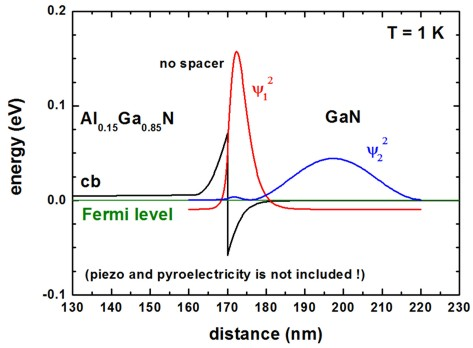Into the equation for the deformation potential acoustic phonon scattering, the quantum well width is an input parameter. We used values which correspond roughly to the extension of the ground state wave function inside the "triangular" QW. ```quantum-well-width = 15d0 ! 15 [nm]``` ```quantum-well-width = 10d0 ! 10 [nm]``` 2DEG sheet density 2DEG sheet density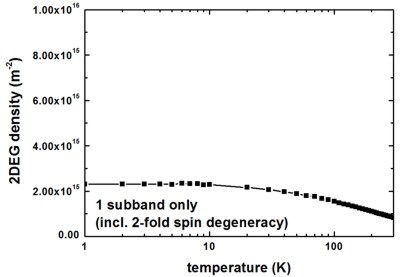[WalukiewiczGaN] used a 2DEG density of 6.2 * 1015 m-2. [WalukiewiczGaN] used a 2DEG density of 1.59 * 1016 m-2. Mobility Mobility ``` impurity-background-doping-concentration = 1d14 ! [cm-3] remote-doping-sheet-density       = 7.883735d11 ! [cm-2]  ```[WalukiewiczGaN] Fig. 4: 7 * 1017 [cm-3]``` ==> ```7 * 1017 [cm-3]2/3 ``` impurity-background-doping-concentration = 4d15 ! [cm-3] remote-doping-sheet-density              = 1d12 ! [cm-2]  ```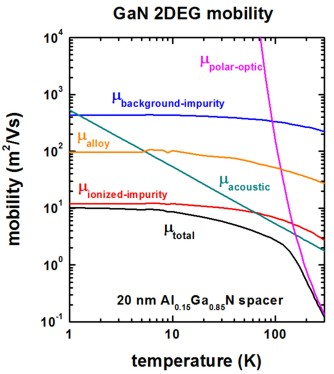``` alloy-disorder-scattering-potential = 2.3d0 ! [eV] ```conduction band offset GaN/AlN

The following GaN material parameters have been used:

``` conduction-band-masses         = 0.21d0   0.21d0   0.21d0     ! [m0] [WalukiewiczGaN]  static-dielectric-constants    = 9.5d0    9.5d0    9.5d0      ! [WalukiewiczGaN] epsilon(0)  optical-dielectric-constants   = 5.35d0   5.35d0   5.35d0     ! [WalukiewiczGaN] epsilon(infinity)  LO-phonon-energy               = 0.0905d0 0.0905d0 0.0905d0   ! [eV] [WalukiewiczGaN] (optical phonon energy)  mass-density                   = 6.1d3                        ! [kg/m^3]  [WalukiewiczGaN]  sound-velocity                 = 6.6d3                        ! [m/s]     [WalukiewiczGaN]  acoustic-deformation-potential = 8.5d0                        ! [eV]      [WalukiewiczGaN]```

Here, alloy scattering is only relevant for the part of the wave function that penetrates into the AlGaN barrier.

Final remark: In principle, the results of these GaN 2DEGs are not reliable as piezo- and pyroelectricity have to be included.
Our results disagree quantitatively with the results of [WalukiewiczGaN]. However, it is not clear, which material parametes he used for the conduction band offset and the alloy scattering.

## Further hints

If two remote doping regions should be taken into account, one can input an array of values.
``` spacer-width                          = 20d0   10d0   ! [nm]   ``` spacer width of 1st and 2nd doping region``` remote-doping-sheet-density           = 1d12   1d11   ! [cm-2] ``` remote doing density of 1st and 2nd doping region

• Please help us to improve our tutorial. Send comments to``` support [at] nextnano.com```.